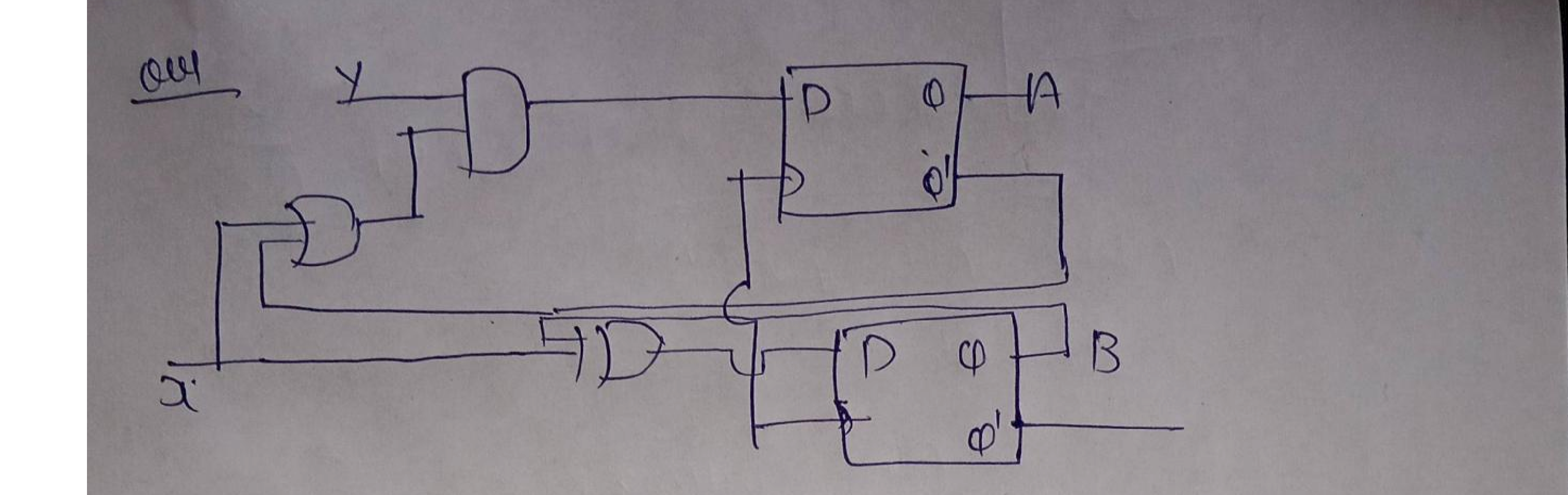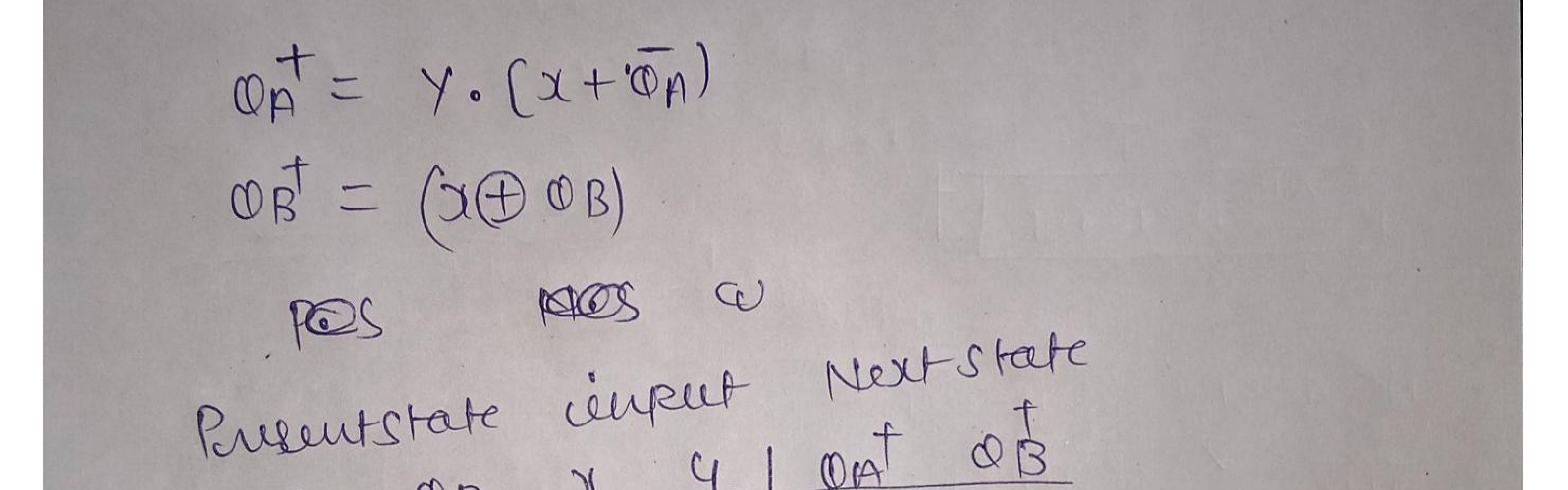Question

Communications

Question 3 (Sequential Logic): Construct the state equations and the state table for the following circuit. Plot the state diagram.For the following X(t) and Y(t) inputs, draw A(t) and B(t), where the initial values of A(t) and B(t) are 0.Verified### Question 44968Communications

\text { For the complex vector } z=\left[\begin{array}{l} z_{1} \\ z_{2} \end{array}\right] \text { in the previous question }
[2-а.What is the PDF, mean and covariance matrix of the vector q = U z where U is a deterministic(constant) unitary matrix. Unitary matrix is a matrix that has the property U" U = I
b. In part (a), did you find out that the two vectors q and z have the same PDF? If Yes, we call zisotropic vector. Read through the Summary Box (Summary A1) in Appendix A

### Question 44967Communications

Now assume two independent complex random variables z, and z. Each one of them is Gaussian withzero mean and variance o² = 1
\text { a. What is the mean vector and covariance matrix of the vector } z=\left[\begin{array}{l} z_{1} \\ z_{2} \end{array}\right]
b. What is the mean (expectation) of y = eJ® zwhere ele is a complex exponential with aconstant (deterministic) angle 0
d. In part (c), if the correct answer is (i) then the vector z is called circular symmetric vector. If the correct answer is (ii) then the vector z is not circular symmetric vector. Read through the Appendix A to learn more about this statement.
\text { i. } E(y)=e^{j \theta} E(z)
\text { ii. } E(V) \neq e^{i \theta} E(z)
In part (b), you calculated E(y). Which one of the following is correct:

### Question 44966Communications

Now assume two independent real random variables x, and x2. Each one of them is Gaussian with zeromean and variance o2 = 1
\text { a. What is the mean vector and covariance matrix of the vector } x=\left[\begin{array}{l} x_{1} \\ x_{2} \end{array}\right]
b. What is the mean vector and covariance matrix of the vector z = Ax + b where A is a 2x2deterministic (constant) matrix and b is a 2x1 deterministic (constant) vector.

### Question 44965Communications

Assume x is a real Gaussian random variable with zero mean and o? variance
Write the probability density function (PDF) of the x
b. Write the PDF of the random variable y = a x + b where a and b are two deterministic real values.

### Question 44311Communications

The message signal m(t) has the Fourier transform shown in Figure P-3.11(a). This signal is applied to the system shown in Figure P-3.11(b) to generate the signal y(t).
1. Plot Y (f), the Fourier transform of y(t).
2. Show that if y(t) is transmitted, the receiver can pass it through a replica of the system shown in Figure P-3.11 (b) to obtain m(t) back. This means that this system can be used as a simple scrambler to enhance communication privacy.

### Question 44310Communications

The message signal m(t), whose spectrum is shown in Figure P-3.18, is passedthrough the system shown in that figure.
The bandpass filter has a bandwidth of 2W centered at fo, and the lowpass filter has a bandwidth of W. Plot the spectra of the signals x (t), yı (t), y2(t), y3 (t), and y4 (1).What are the bandwidths of these signals?

### Question 44309Communications

A DSB-SC AM signal is modulated by the signal
m(t)=2 \cos 2000 \pi t+\cos 6000 \pi t .
The modulated signal is
u(t)=100 m(t) \cos 2 \pi f_{c} t
\text { where } f_{c}=1 \mathrm{MHz}
1. Determine and sketch the spectrum of the AM signal.
2. Determine the average power in the frequency components.

### Question 44308Communications

The message signal m(t) = 2 cos 400t + 4 sin(500r +) modulates the carrier signal c(t) = A cos(8000 rt), using DSB amplitude modulation. Find the time-domain and frequency-domain representations of the modulated signal and plot the spectrum (Fourier transform) of the modulated signal. What is the power content of the modulated signal?

### Question 44307Communications

\text { Prove that the power spectrum density of the output } y(t) \text { is } S_{y}(f)=S_{x}(f)|H(f)|^{2}

### Question 44064Communications

\text { For the complex vector } z=\left[\begin{array}{l} z_{1} \\ z_{2} \end{array}\right] \text { in the previous question }
a. What is the PDF, mean and covariance matrix of the vector g = U z where U is a deterministic(constant) unitary matrix. Unitary matrix is a matrix that has the property U"U = I
b. In part (a), did you find out that the two vectors q and z have the same PDF? If Yes, we call zisotropic vector. Read through the Summary Box (Summary A1) in Appendix A

### Submit query